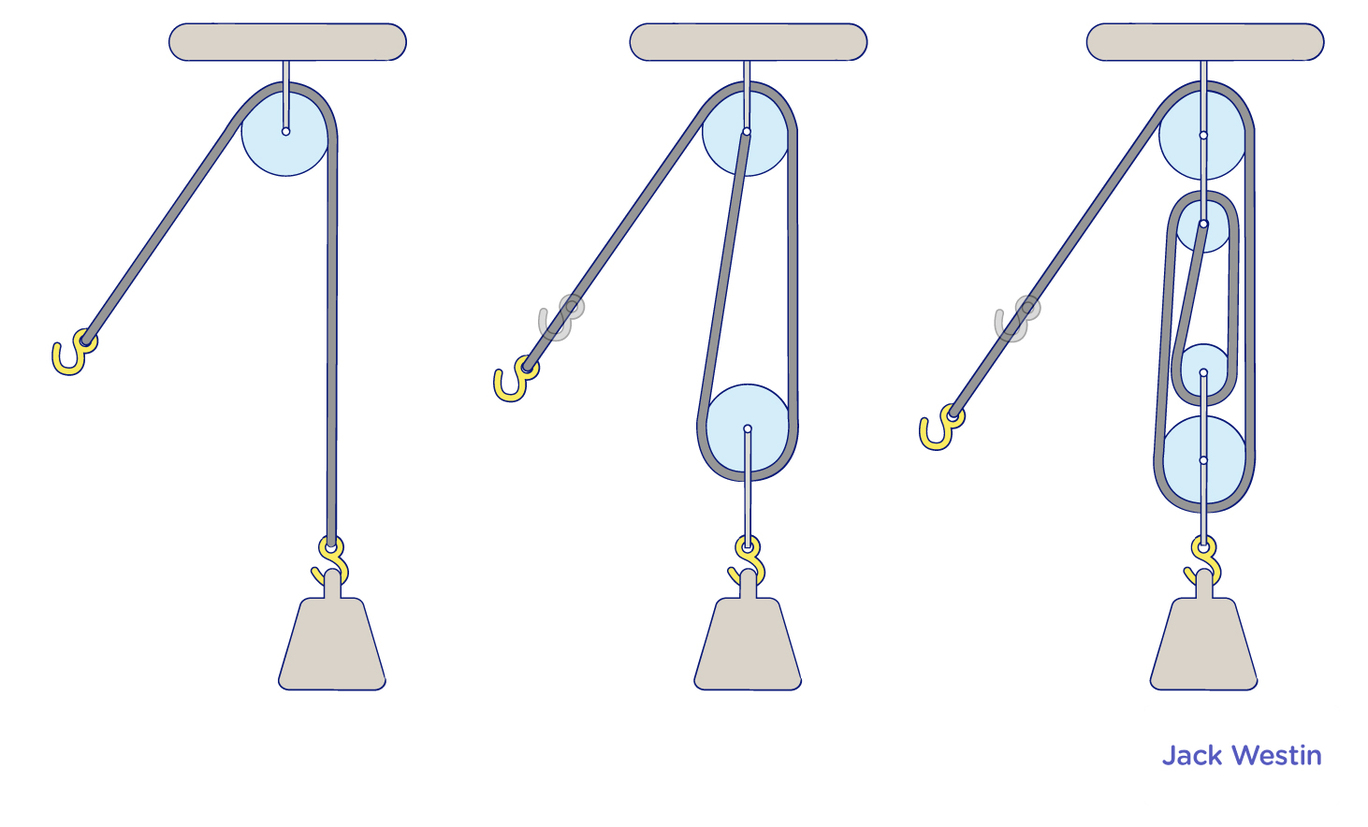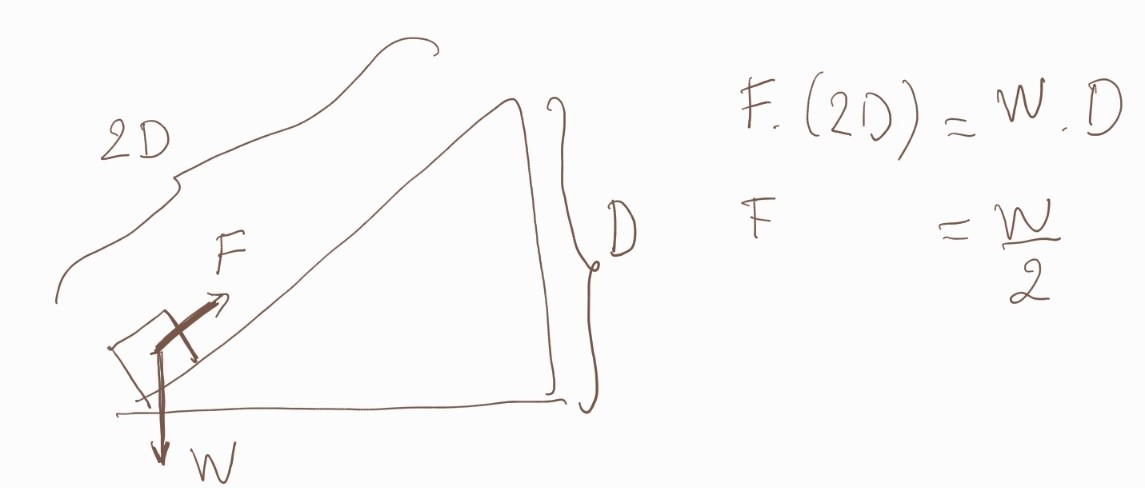Cyber Sale - Only 3 Days Remaining: ALL MCAT Products & Admissions Services

MCAT Content / Work / Mechanical Advantage

Topic: Work

Mechanical advantage is a measure of the ratio of output force to input force in a system, used to analyze the forces in simple machines like levers and pulleysWhen forces on an object change the amount of energy output from a system is still the same as the input due to the conservation of energy. Typically the mechanical advantage is expressed in ideal terms, where there are no losses in energy between the input and output times (no heat, friction loss), also known as 100% efficient systems.The left side is the input energy (or work put into a machine – seesaw), and the right side is the output energy (or work that comes out of a machine, for no-loss energy exchange).

Once you know the ratio of the displacements (d), you can find the relationship between the 2 forces.Key Points

• We can find the ratio of output force to input force considering Work in equals to Work out.

• Mechanical advantage is expressed in ideal terms, no loss of energy to heat for friction.

Key Terms

simple machines: a mechanical device that changes the direction or magnitude of a force like a wedge, levers, pulleys

mechanical advantage: a measure of the ratio of output force to input force in a system

Billing Information